## 10.2.2 Policy Iteration

The value iterations of Section 10.2.1 work by iteratively updating cost-to-go values on the state space. The optimal plan can alternatively be obtained by iteratively searching in the space of plans. This leads to a method called policy iteration ; the term policy is synonymous with plan. The method will be explained for the case of probabilistic uncertainty, and it is assumed thatis finite. With minor adaptations, a version for nondeterministic uncertainty can also be developed.

Policy iteration repeatedly requires computing the cost-to-go for a given plan,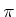. Recall the definition of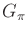from (10.32). First suppose that there are no uncertainties, and that the state transition equation is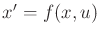. The dynamic programming equation (2.18) from Section 2.3.2 can be used to derive the cost-to-go for each state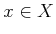under the application of. Make a copy of (2.18) for each, and instead of the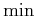, use the given action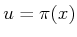, to yield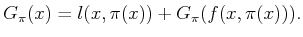(10.50)

In (10.50), the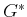has been replaced bybecause there are no variables to optimize (it is simply the cost of applying). Equation (10.50) can be thought of as a trivial form of dynamic programming in which the choice of possible plans has been restricted to a single plan,. If the dynamic programming recurrence (2.18) holds over the space of all plans, it must certainly hold over a space that consists of a single plan; this is reflected in (10.50).

If there arestates, (10.50) yieldsequations, each of which gives an expression of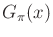for a different state. For the states in which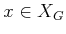, it is known that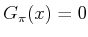. Now that this is known, the cost-to-go for all states from which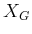can be reached in one stage can be computed using (10.50) with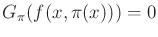. Once these cost-to-go values are computed, another wave of values can be computed from states that can reach these in one stage. This process continues until the cost-to-go values are computed for all states. This is similar to the behavior of Dijkstra's algorithm.

This process of determining the cost-to-go should not seem too mysterious. Equation (10.50) indicates how the costs differ between neighboring states in the state transition graph. Since all of the differences are specified and an initial condition is given for, all others can be derived by adding up the differences expressed in (10.50). Similar ideas appear in the Hamilton-Jacobi-Bellman equation and Pontryagin's minimum principle, which are covered in Section 15.2.

Now we turn to the case in which there are probabilistic uncertainties. The probabilistic analog of (2.18) is (10.49). For simplicity, consider the special case in which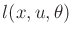does not depend on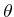, which results in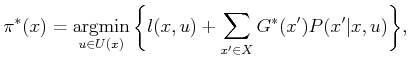(10.51)

in which. The cost-to-go function,, satisfies the dynamic programming recurrence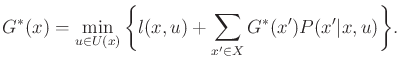(10.52)

The probabilistic analog to (10.50) can be made from (10.52) by restricting the set of actions to a single plan,, to obtain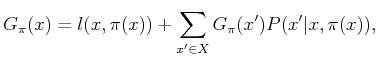(10.53)

in which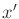is the next state.

The cost-to-go for eachunder the application ofcan be determined by writing (10.53) for each state. Note that all quantities exceptare known. This means that if there arestates, then there arelinear equations andunknowns (for each). The same was true when (10.50) was used, except the equations were much simpler. In the probabilistic setting, a system oflinear equations must be solved to determine. This may be performed using classical linear algebra techniques, such as singular value decomposition (SVD) [399,961].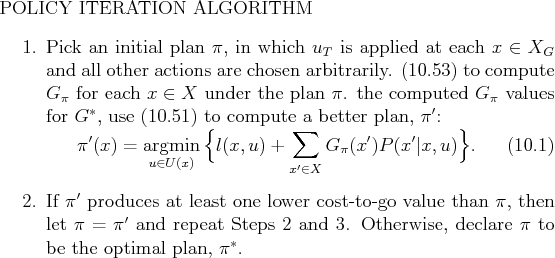Now that we have a method for evaluating the cost of a plan, the policy iteration method is straightforward, as specified in Figure 10.4. Note that in Step 3, the cost-to-go, which was developed for one plan,, is used to evaluate other plans. The result is the cost that will be obtained if a new action is tried in the first stage and thenis used for all remaining stages. If a new action cannot reduce the cost, thenmust have already been optimal because it means that (10.54) has become equivalent to the stationary dynamic programming equation, (10.49). If it is possible to improve, then a new plan is obtained. The new plan must be strictly better than the previous plan, and there is only a finite number of possible plans in total. Therefore, the policy iteration method converges after a finite number of iterations.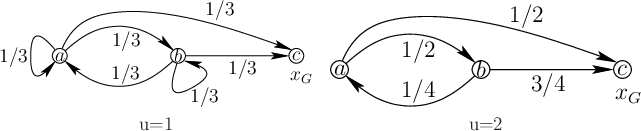Example 10..7 (An Illustration of Policy Iteration)   A simple example will now be used to illustrate policy iteration. Let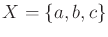and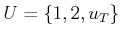. Let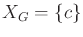. Let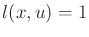for alland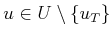(if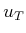is applied, there is no cost). The probabilistic state transition graphs for each action are shown in Figure 10.5. The first step is to pick an initial plan. Let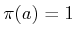and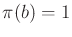; let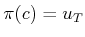because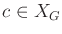.

Now use (10.53) to compute. This yields three equations: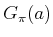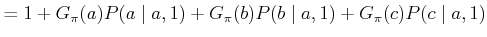(10.54)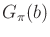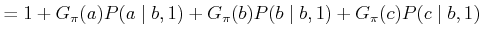(10.55)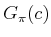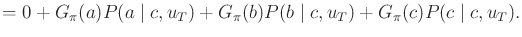(10.56)

Each equation represents a different state and uses the appropriate action from. The final equation reduces to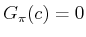because of the basic rules of applying a termination condition. After substituting values for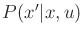and using, the other two equations become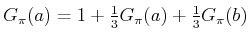(10.57)

and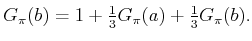(10.58)

The solutions are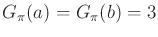.

Now use (10.54) for each state withandto find a better plan,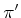. At state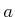, it is found by solving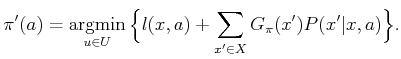(10.59)

The best action is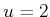, which produces cost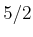and is computed as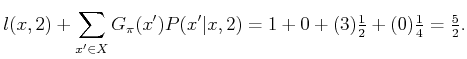(10.60)

Thus,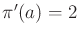. Similarly,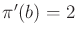can be computed, which produces cost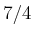. Once again,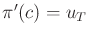, which completes the determination of an improved plan.

Since an improved plan has been found, replacewithand return to Step 2. The new plan yields the equations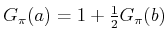(10.61)

and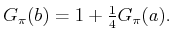(10.62)

Solving these yields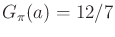and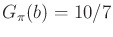. The next step attempts to find a better plan using (10.54), but it is determined that the current plan cannot be improved. The policy iteration method terminates by correctly reporting that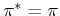.Policy iteration may appear preferable to value iteration, especially because it usually converges in fewer iterations than value iteration. The equation solving that determines the cost of a plan effectively considers multiple stages at once. However, for most planning problems,is large and the large linear system of equations that must be solved at every iteration can become unwieldy. In some applications, either the state space may be small enough or sparse matrix techniques may allow efficient solutions over larger state spaces. In general, value-based methods seem preferable for most planning problems.

Steven M LaValle 2020-08-14